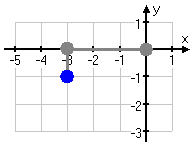Plottings Points in the Cartesian Plane (page 2 of 3)

Sections: Introduction to the plane, Plotting points, The four quadrantsWhen you were trying to find your street on that map, you went over to D and then down to 12. And that "D12" designation was unambiguous, because it was easy to tell which stood for which. Even if the designation had been written as "12-D", you still would have known which box to go to, because the "D" would still have been across the top and the "12" would still have been along the side. But in the Cartesian plane, both axes are labelled with numbers. How can you tell how far left or right to go, or how far up or down to go?

Suppose you were told to locate "(5, 2)" (pronounced as "the point five two" or just "five two") on the plane. Where would you look? To understand the meaning of "(5, 2)", you have to know the following rule: The x-coordinate (the number for the x-axis) always comes first. The first number (the first coordinate) is always on the horizontal axis.

 So, for the point (5, 2), you would start at the "origin", the spot where the axes cross: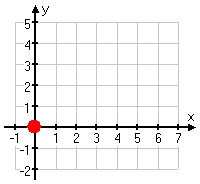...then count over to "five" on the x-axis: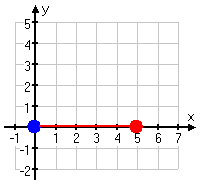...then count up to "two", moving parallel to the y-axis: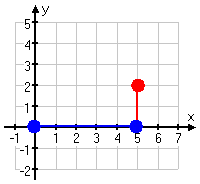...and then draw in the dot: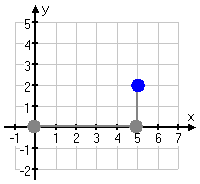Finding the location of (5, 2) and then drawing its dot is called "plotting the point (5, 2)".

When plotting, remember that the first number is for the horizontal axis and the second number is for the vertical axis. You always go "so far over or back" and then "so far up or down".

• Plot the point (4, –5).
 I will start at the origin: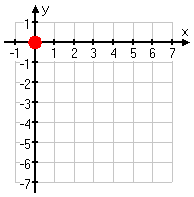...then I'll count over four units on the horizontal x-axis: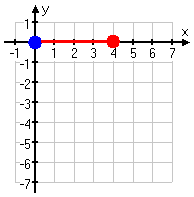...then I'll count down five units paralell to the y-axis: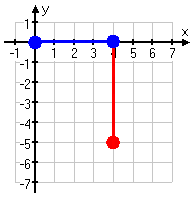...and then I'll draw my dot: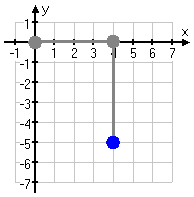As you can see above, a negative y-coordinate means that you'll be counting down the y-axis, not up.

 I'll start at the origin: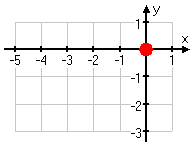...then I'll count backwards three units along the x-axis: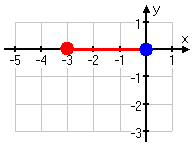...then I'll count down one unit parallel to the y-axis: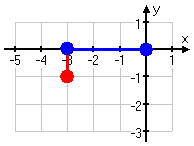...and then I'll draw my dot: